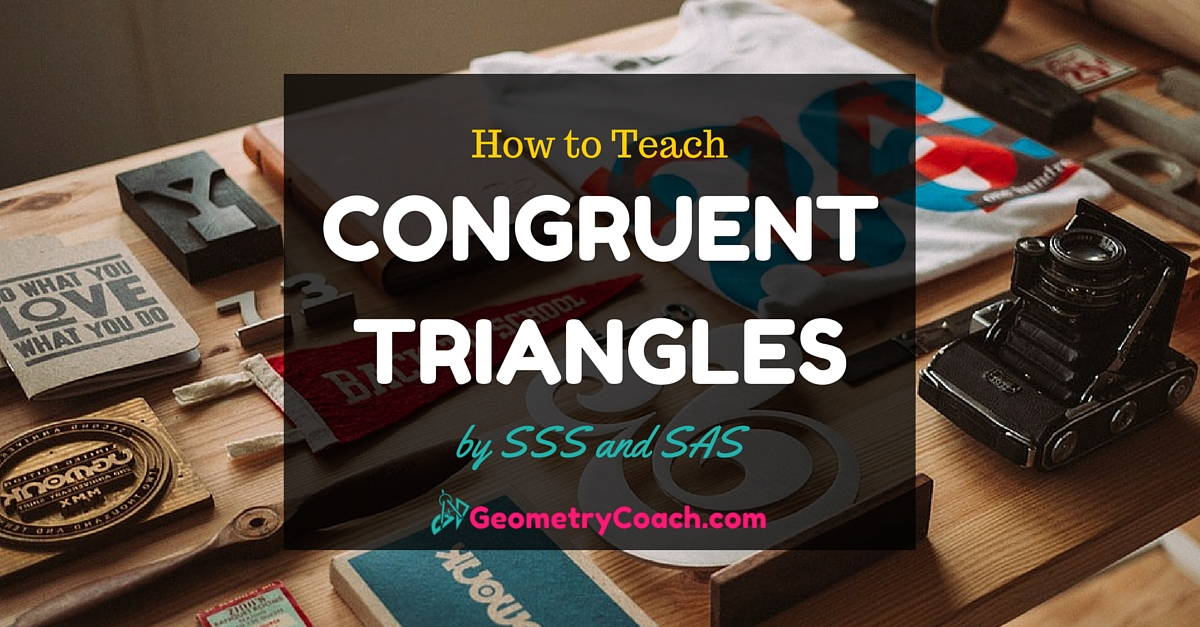# Triangle Congruence by SSS and SAS

Before you can ever start with proofs your students need to have a clear understanding of what makes sides and angles of triangles congruent. This lesson on Triangle Congruence by SSS and SAS is one of the more memorization based lessons to teach. With that said the only way to memorize something and master is it to see it multiple times in multiple ways. Here are a couple of things to help you out!

## Congruent Figures

Two figures are said to be congruent if they have same shape and the same size. The corresponding angles of the two congruent figures are equal. The corresponding sides are congruent.
The two figures shown below are congruent.The sides of two rectangles have a ratio of 1: 1. The rectangles are congruent.

## Congruent Triangles

### Triangle Congruence by Side Side Side

The congruence of triangles can be proved using its three sides and three angles.

#### If all the three sides of one triangle are congruent to all the three corresponding sides of another triangle, the two triangles are said to be congruent.

The two triangles shown below are congruent by SSS Postulate.### Triangle Congruence by Side Angle Side

#### If the two sides and their included angle of one triangle is congruent to the two sides and their included angle of another triangle, then the two triangles are said to be congruent.

The included angle is the angle made at the point where two sides of a triangle meet. The two triangles shown below are congruent by SAS Postulate.## Triangle Congruence by SSS and SAS Worksheet – Docs & PowerPoint

To gain access to our editable content Join the Geometry Teacher Community!
Here you will find hundreds of lessons, a community of teachers for support, and materials that are always up to date with the latest standards.

## Triangle Congruence by SSS and SAS – PDFs

4-2 Assignment (FREEBIE)

4-2 Bell Work (FREEBIE)

4-2 Exit Quiz (FREEBIE)

4-2 Guided Notes SE (FREEBIE)

4-2 Guided Notes – Teacher Edition  (Members Only)

4-2 Lesson Plan  (Members Only)

4-2 Online Activities (Members Only)

4-2 Slide Show (FREEBIE)